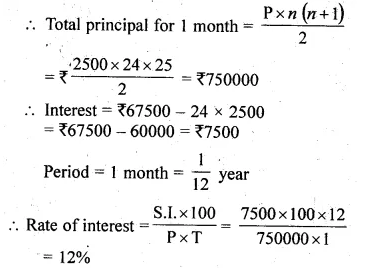Guru

# Mr. Gupta-opened a recurring deposit account in a bank. He deposited Rs. 2500 per month for two years. At the time of maturity he got Rs. 67500. Find : (i) the total interest earned by Mr. Gupta. (ii) the rate of interest per annum.

• 0

This is an important question from the Book- ML Aggarwal
Board- ICSE
Publication- Avichal
Chapter- Banking
Chapter number-2
This question has been asked in 2010 questions paper.

Mr. Gupta-opened a recurring deposit account in a bank. He deposited Rs. 2500 per month for two years. At the time of maturity he got Rs. 67500.

Find : (i) the total interest earned by Mr. Gupta.

(ii) the rate of interest per annum.

Question no.7 , ML Aggarwal, exercise 2, chapter 2, ICSE board, class10, banking.

Share